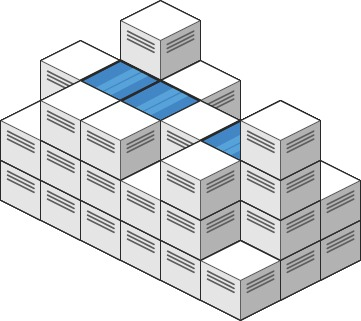# Leetcode 407 - Trapping water II

Note:

• How many water can a tile contains depend on max(itself, shortest neighbor).
• Start from the outermost tile. Put them one by one in a Min-heap priority queue, and mark them true in visited[].
• Each time pop the shortest pillar from PQ, and check all its neighbors.
• If it’s higher than some of its neighbors
• Fill the neighbor up.
• Put them in the PQ.
• Mark as visited
• res = curHeight - neighbor's height.
• Terminate when the queue is empty.

Question:

Given an m x n integer matrix heightMap representing the height of each unit cell in a 2D elevation map, return the volume of water it can trap after raining.

Example:Code: